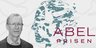# The Abel Prize 2016 to Andrew Wiles for his proof of Fermat's Last Theorem

Condividi su
J. Kramer    30-05-2016     Leggi in PDFCredits: photo Alain Goriely/Mathematical Institute, University of Oxford.

The Norwegian Academy of Science and Letters has decided to award the Abel Prize for 2016 to Sir Andrew J. Wiles (University of Oxford) "for his stunning proof of Fermat's Last Theorem by way of the modularity conjecture for semistable elliptic curves, opening a new era in number theory".

Number theory, an old and beautiful branch of mathematics, is concerned with the study of arithmetic properties of the integers. In its modern form the subject is fundamentally connected to various fields of mathematics. Number theoretic results play an important role in our everyday lives through encryption algorithms for communications, financial transactions, and digital security.

Fermat's Last Theorem, first formulated by Pierre de Fermat in the 17th century, is the assertion that the equation xn+yn=zn has no solutions in positive integers for n>2. Fermat proved his claim for n=4, Leonhard Euler found a proof for n=3, and Sophie Germain proved the first general result that applies to infinitely many prime exponents. Ernst Kummer's study of the problem unveiled several basic notions in algebraic number theory, such as ideal numbers and the subtleties of unique factorization. The complete proof found by Andrew Wiles relies on three further concepts in number theory, namely elliptic curves, modular forms, and Galois representations.

Elliptic curves are defined by cubic equations in two variables. They are the natural domains of definition of the elliptic functions introduced by Niels Henrik Abel. Modular forms are highly symmetric analytic functions defined on the upper half of the complex plane, and naturally factor through shapes known as modular curves. An elliptic curve is said to be modular if it can be parametrized by a map from one of these modular curves. The modularity conjecture, proposed by Goro Shimura, Yutaka Taniyama, and André Weil in the 1950s and 60s, claims that every elliptic curve defined over the rational numbers is modular.

In 1984, Gerhard Frey associated a semistable elliptic curve to any hypothetical counterexample to Fermat's Last Theorem, and strongly suspected that this elliptic curve would not be modular. Frey's non-modularity was proven via Jean-Pierre Serre's epsilon conjecture by Kenneth Ribet in 1986. Hence, a proof of the Shimura-Taniyama-Weil modularity conjecture for semistable elliptic curves would also yield a proof of Fermat's Last Theorem. However, at the time the modularity conjecture was widely believed to be completely inaccessible. It was therefore a stunning advance when Andrew Wiles, in a breakthrough paper published in 1995, introduced his modularity lifting technique and proved the semistable case of the modularity conjecture.

The modularity lifting technique of Wiles concerns the Galois symmetries of the points of finite order in the abelian group structure on an elliptic curve. Building upon Barry Mazur's deformation theory for such Galois representations, Wiles identified a numerical criterion which ensures that modularity for points of order p can be lifted to modularity for points of order any power of p, where p is an odd prime. This lifted modularity is then sufficient to prove that the elliptic curve is modular. The numerical criterion was confirmed in the semistable case by using an important companion paper written jointly with Richard Taylor. Theorems of Robert Langlands and Jerrold Tunnell show that in many cases the Galois representation given by the points of order three is modular. By an ingenious switch from one prime to another, Wiles showed that in the remaining cases the Galois representation given by the points of order five is modular. This completed his proof of the modularity conjecture, and thus also of Fermat's Last Theorem.